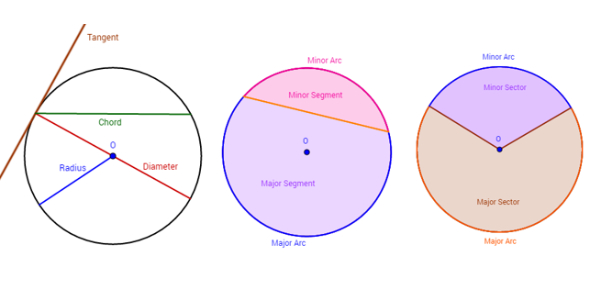# Identify The Different Parts Of A Circle

5 Questions | Total Attempts: 6468SettingsYes, the circle has parts! A circle may seem the same at all points but the quiz below will enlighten you on identifying the parts of a circle. It is a challenge worth a shot. Enjoy the discovery!

• 1.
Which of the following is a chord, but not a diameter?
• A.

PR

• B.

QR

• C.

PT

• 2.
Which of the following is a radius?
• A.

PQ

• B.

QR

• C.

QS

• D.

All of the above

• 3.
Name the center of this circle.
• A.

Point P

• B.

Point Q

• C.

Point T

• D.

Point R

• 4.
What is PR (or PQR)?
• A.

Diameter

• B.

• C.

Chord

• D.

Arc

• 5.
If PQ is 3 cm long, then how long is PR?
• A.

1.5 cm

• B.

12 cm

• C.

6 cm

• D.

3 cm

Related TopicsBack to top